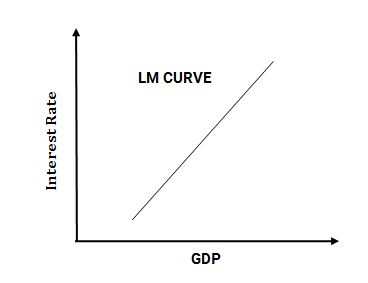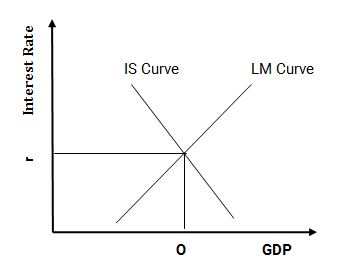# The IS-LM Model

IS (Interest, Saving) and LM (Liquidity, Money Supply model is an economic model that shows the interaction between Goods and Financial Market. The IS curve is related to the Goods Market and the LM curve is related to the Financial Market. IS-LM Model is also known as the Hicks-Hansen Model.

## IS Curve

• With the increase in the interest rate in an economy, people tend to hold money in the form of Savings and when the interest rate declines, people spend the money. Interest/Saving Curve is obtained when the graph of Interest Rate vs GD is plotted as shown below.

## LM Curve

• With the Increase in Interest Rate, people tend to save/invest more, hence the liquidity in the Financial Market increases, people buy more and more savings and funds flow in the Financial Market. Interest/Saving Curve is obtained when the graph of Interest Rate vs GD is plotted as shown below.
• The LM curve is upward sloping because with the increase in interest rate, the liquidity in financial markets increases, this liquidity is utilized in growth/production-related activities that increase the GDP, hence at high-interest rates both GDP and liquidity are high.## IS-LM Curve

Upon merging the above two graphs, we get the following graph:• The point of intersection of the IS/LM Curve is the Equilibrium point of Interest Rate and GDP. The intersection of the IS and LM curves shows the equilibrium point of interest rates and output when money markets and the real economy are in balance.